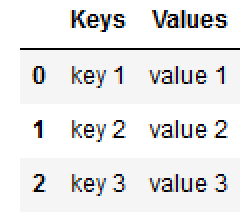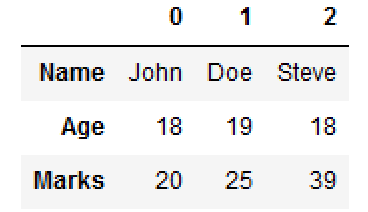Data Science

# Convert Python dict to Pandas Dataframe

Converting a Python dictionary to a Pandas DataFrame is a fundamental data manipulation task, as it enables data professionals to use Pandas' powerful data structures and functionalities for analysis and visualization. The process involves transforming the key-value pairs of a dictionary into columns and rows of a DataFrame, effectively organizing the data in a tabular format.

Pandas provides a convenient function called pd.DataFrame() that can be used for this conversion. Let's explore how to convert a Python dictionary to a Pandas DataFrame with examples:

## Simple dictionary with lists as values

Suppose we have a Python dictionary containing information about students:

import pandas as pd data = { 'Name': ['John', 'Billy', 'Smith', 'David'], 'Age': [25, 30, 22, 28], 'Score': [90, 85, 95, 88] } # Convert the dictionary to a Pandas DataFrame df = pd.DataFrame(data) print(df)
Output: Name Age Score 0 John 25 90 1 Billy 30 85 2 Smith 22 95 3 David 28 88

In this example, the Python dictionary data is converted into a Pandas DataFrame df. Each key in the dictionary becomes a column, and the corresponding lists as values populate the rows of the DataFrame.

## Dictionary with nested dictionaries as values

Suppose we have a Python dictionary containing information about students with additional details:

import pandas as pd data = { 'Name': ['John', 'Billy', 'Smith', 'David'], 'Details': { 'Age': [25, 30, 22, 28], 'Score': [90, 85, 95, 88] } } # Convert the dictionary to a Pandas DataFrame df = pd.DataFrame(data) print(df)
Output: Name Details 0 John {'Age': 25, 'Score': 90} 1 Billy {'Age': 30, 'Score': 85} 2 Smith {'Age': 22, 'Score': 95} 3 David {'Age': 28, 'Score': 88}

In this example, the Python dictionary data contains a nested dictionary with details for each student. When converted to a Pandas DataFrame, the 'Details' key becomes a column containing dictionaries as values.

## Dictionary Keys and Values as DataFrame rows

myDict = {'key 1': 'value 1', 'key 2': 'value 2', 'key 3': 'value 3'} df = pd.DataFrame.from_dict(myDict.items()) df.columns = ['Keys', 'Values'] dfKeys Values 0 key 1 value 1 1 key 2 value 2 2 key 3 value 3

## Dictionary Keys and Values as DataFrame rows

myDict = {'key 1': 'value 1', 'key 2': 'value 2', 'key 3': 'value 3'} pd.DataFrame({'Keys' : myDict.keys() , 'Values' : myDict.values() })
Keys Values 0 key 1 value 1 1 key 2 value 2 2 key 3 value 3

## Keys to DataFrame Column and Values to DataFrame Rows

myDict = {'key 1': 'value 1', 'key 2': 'value 2', 'key 3': 'value 3'} df = pd.DataFrame([myDict], columns=myDict.keys()) df
key 1 key 2 key 3 0 value 1 value 2 value 3

## Keys to DataFrame Column and Values to DataFrame Rows

df = pd.DataFrame({'Item': ['Item_1', 'Item_2', 'Item_3'], 'Price': [200, 400, 100]}) df
Item Price 0 Item_1 200 1 Item_2 400 2 Item_3 100

## Keys to DataFrame Column and Values to DataFrame Rows

dict = [{'Name': 'John', 'Age': 18, 'Marks': 20}, {'Name': 'Doe', 'Age': 19, 'Marks': 25}, {'Name': 'Steve', 'Age': 18, 'Marks': 39 }] df = pd.DataFrame.from_dict(dict) df
Name Age Marks 0 John 18 20 1 Doe 19 25 2 Steve 18 39

## Keys to DataFrame Rows and Values to DataFrame columns

dict ={'Name': ['John', 'Doe', 'Steve'], 'Age':[18, 19, 18], 'Marks':[20, 25, 39]} df = pd.DataFrame.from_dict(dict, orient ='index') df0 1 2 Name John Doe Steve Age 18 19 18 Marks 20 25 39

Pandas automatically aligns data in a tabular format while converting a dictionary to a DataFrame, and it also handles missing values appropriately.

### Conclusion

In conclusion, the process of converting a Python dictionary to a Pandas DataFrame is a seamless and versatile operation that enhances the data exploration and analysis capabilities of Python. By utilizing the power of Pandas, data professionals can manipulate, visualize, and derive insights from data with elegance and precision. The DataFrame structure, fortified by the essence of Python dictionaries, stands as a cornerstone in the data science landscape, elevating Python's position as a dominant force in data-driven knowledge.